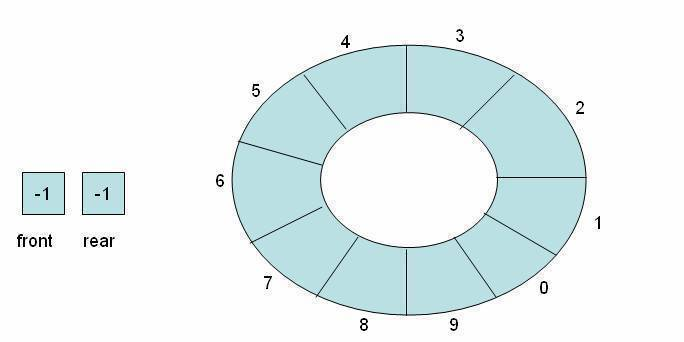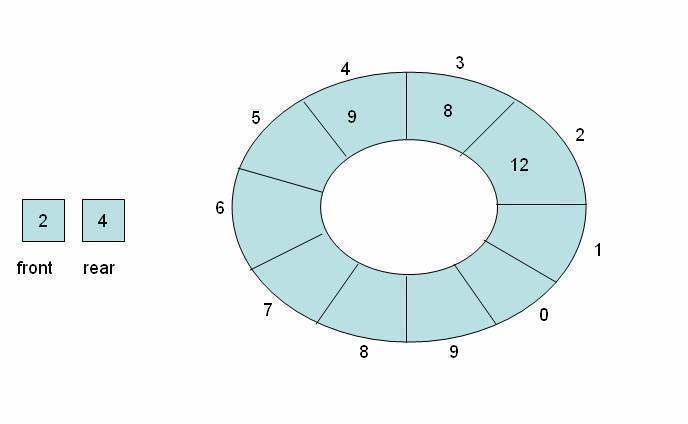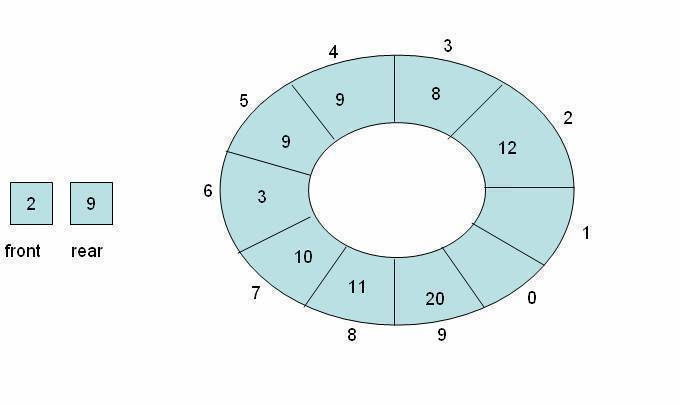•• # Circular Queue

The difficulty of managing front and rear in an array-based non-circular queue can be overcome if we treat the queue position with index 0 as if it comes after the last position (in our case, index 9), i.e., we treat the queue as circular. Note that we use the same array declaration of the queue.

## Empty queue:## Non-empty queues:Implementation of operations on a circular queue:

## Testing a circular queue for overflow

There are two conditions:

• (front=0) and (rear=capacity-1)
• front=rear+1

If any of these two conditions is satisfied, it means that circular queue is full.

.cf { font-family: Lucida Console; font-size: 9pt; color: black; background: white; } .cl { margin: 0px; } .cb1 { color: green; } .cb2 { color: blue; }

`bool isfull(queue *q)`
`{`
` if (((q->front==0)&&( q->rear==CAPACITY-1))||( q->front==q->rear+1))`
` return true;`
` else`
` return false;`
`}`

Note: bool can be defined as typedef enum {false, true} bool;

## The enqueue Operation on a Circular Queue

There are three scenarios which need to be considered, assuming that the queue is not full:

1. If the queue is empty, then the value of the front and the rear variable will be -1 (i.e., the sentinel value), then both front and rear are set to 0.
2. If the queue is not empty, then the value of the rear will be the index of the last element of the queue, then the rear variable is incremented.
3. If the queue is not full and the value of the rear variable is equal to capacity -1 then rear is set to 0.

`void enqueue(queue *q, int value) /*assumes queue is not full*/`
`{`
` /*adjust rear variable*/`
` if (q->front==-1)`
` q->front=q->rear=0;`
` else if (q->rear==CAPACITY-1)`
` q->rear=0;`
` else`
` q->rear++;`
` /*store element at new rear */`
` q->elements[q->rear]=value;`
`}`

## The dequeue Operation on a Circular Queue

Again, there are three possibilities:

1. If there was only one element in the circular queue, then after the dequeue operation the queue will become empty. This state of the circular queue is reflected by setting the front and rear variables to -1.
2. If the value of the front variable is equal to CAPACITY-1, then set front variable to 0.
3. Ifneither of the above conditions hold, then the front variable is incremented

`int dequeue(queue *q)`
`{ `
` int temp=q->elements[q->front]; /*store the front element in a temporary variable, so that we return this value later */`
` `
` /*adjust front variable */ `
` if (q->front == q->rear)`
` q->front = q->rear=-1;`
` else if (q->front == CAPACITY-1)`
` q->front = 0;`
` else`
` q->front++;`
` `
` return temp; /*return the dequeued element*/`
`}`

1. #### Ravi

24 September, 2012 at 7:39 pm

So this Overflow condition occurs only when we’ve already chosen some capacity (like we are statically assigning some memory & then using) for our queue. & hence two conditions. 1. (front=0) and (rear=capacity-1) 2. front=rear+1

If we are implementing Circular Queue by dynamic allocation of memory then there’s no Overflow condition (provided allocation never fails)

2. #### kibrom

10 December, 2011 at 7:05 pm

I think buzzzzzz on testing for empty and full

3. #### rishi

10 March, 2011 at 6:45 pm

any body knows how to identify front and rear in circular queqe